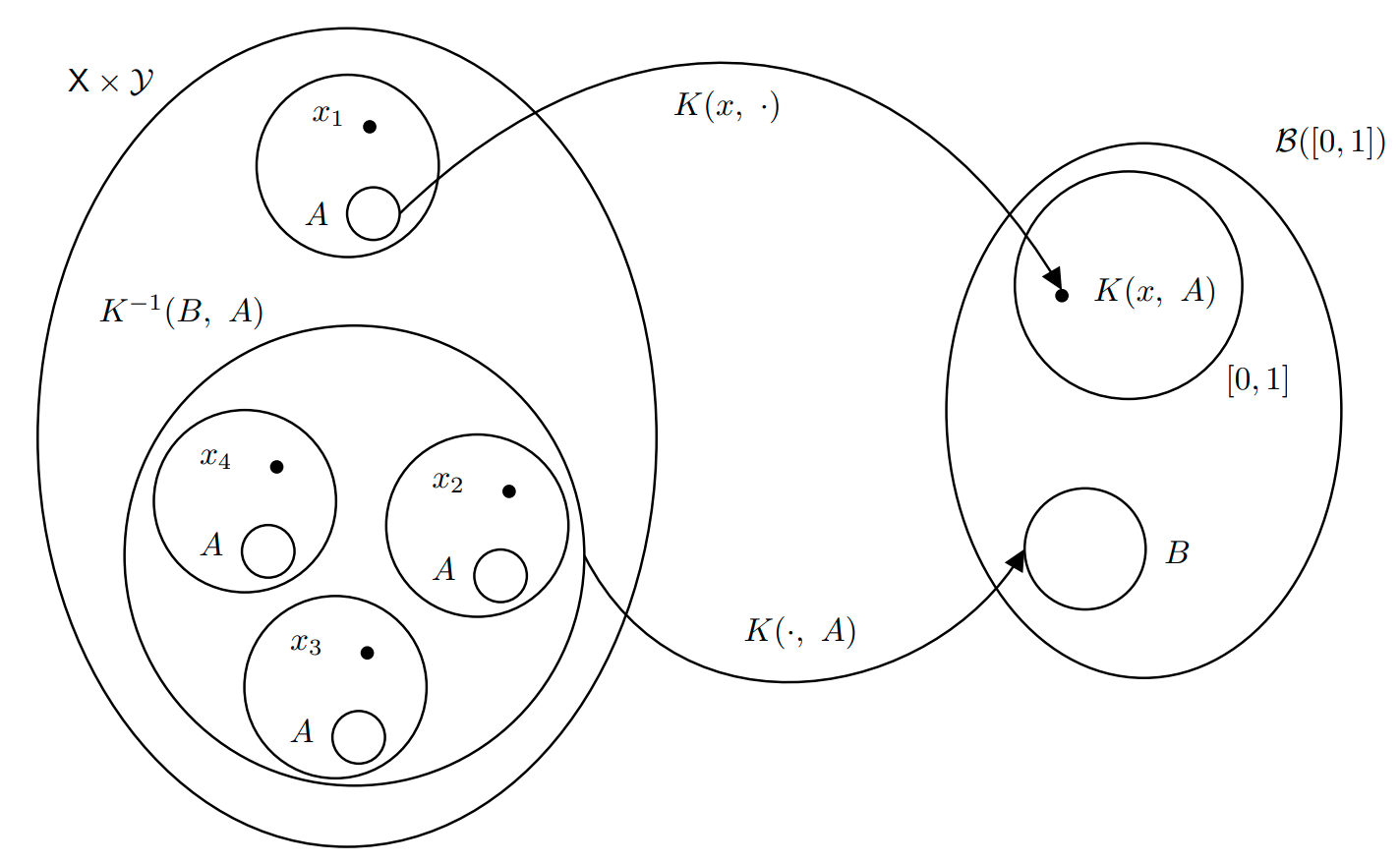# Visualizing Measure Theory for Markov Chains

Visualizing Push-Forward Measures and Transition Kernels with Diagrams

# Sample Space

The sample space $$\Omega$$ is the set of all outcomes of our probabilistic experiment.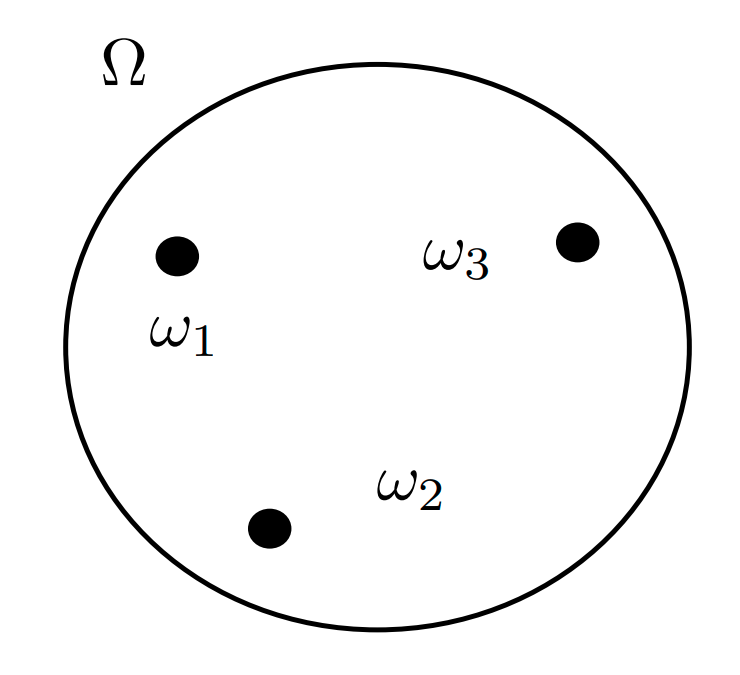For instance:

• Experiment: A single toss of a coin.
• Possible Outcomes: $$\omega_1 = \text{Head}$$ and $$\omega_2 = \text{Tail}$$.
• Sample Space: $$\Omega := \{\omega_1, \omega_2\} = \{\text{Head}, \text{Tail}\}$$

# Event Space

The event space is a sigma-algebra on the sample space. It is a subset of the power set of $$\Omega$$ whose elements, called events, satisfy some regularity conditions. When $$\Omega$$ is finite and discrete, the power set $$\mathcal{P}(\Omega)$$ is a valid sigma algebra that can be used as the event space.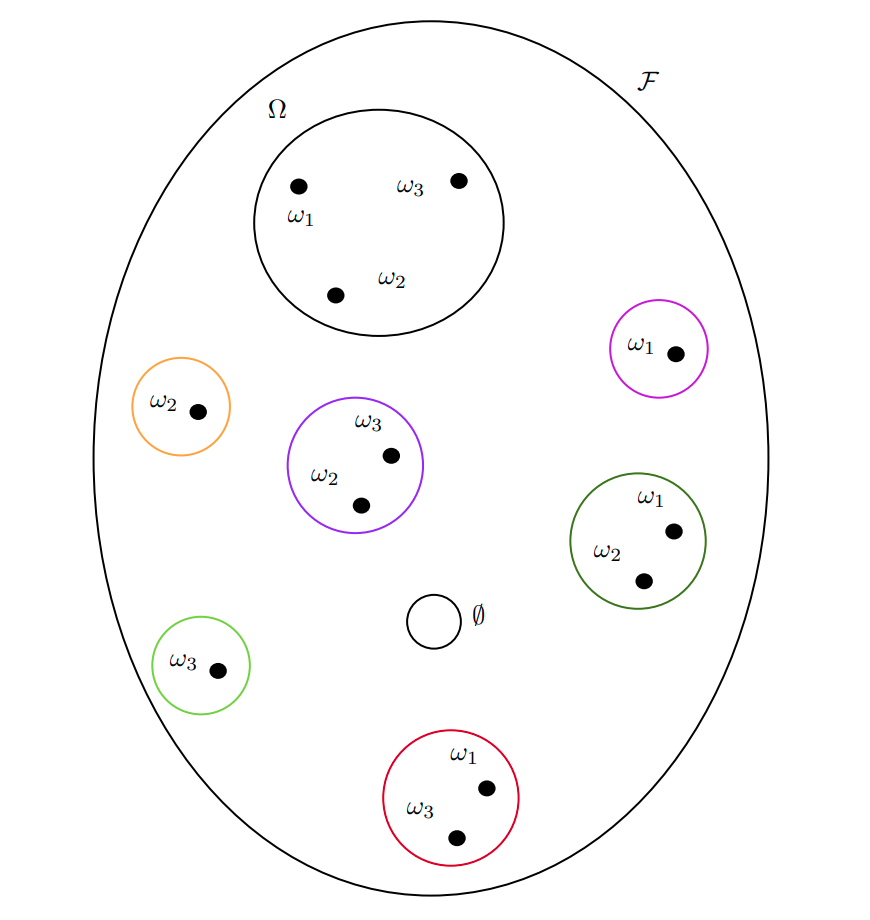Practically $$\mathcal{F}\subseteq \mathcal{P}(\Omega)$$ satisfying

• $$\Omega \in \mathcal{F}$$ The sample space is an event (called the “sure” event).
• $$A\in \mathcal{F} \quad \implies \quad A^c\in\mathcal{F}$$ Closed under complementation.
• $$A_1, A_2, \ldots\in\mathcal{F} \quad \implies \quad \cup_iA_i \in \mathcal{F}$$ Closed under countable unions.

A pair $$(\Omega, \mathcal{F})$$ of a set and its sigma algebra (in this case of the sample space and the event space) is called a measurable space. This is because we can define a function that “measures” the size of the elements of all the elements of $$\mathcal{F}$$. Indeed, any element of a sigma algebra $$B\in\mathcal{F}$$ is called a measurable set.

# Probability Measure and Probability Space

After you’ve defined the sigma-algebra called Event Space, the next step is to define a probability space. A probability space is a triplet $$(\Omega, \mathcal{F}, \mathbb{P})$$, i.e. it is composed of three elements, two of which we already know.

• A sample space $$\Omega$$.
• An event space $$\mathcal{F}$$ which is a sigma-algebra on $$\Omega$$.
• A probability measure $$\mathbb{P}$$.

A probability measure is a special case of a measure. Suppose you have a measurable space $$(\Omega, \mathcal{F})$$ (also referred to as $$\Omega\times\mathcal{F})$$). Then a function $\mathbb{P}: \mathcal{F} \to [0, +\infty)$ is called a measure on $$(\Omega, \mathcal{F})$$ if it gives a size of zero to the empty set and if it is countably additive:

• $$\mathbb{P}(\emptyset) = 0$$
• $$\mathbb{P}\left(\bigcup_i A_i\right) = \sum_i \mathbb{P}(A_i) \qquad \text{for } A_i\in\mathcal{F}$$

Notice how the measure takes elements of $$\mathcal{F}$$ and not elements of the sample space! That is, it maps events not outcomes. A probability measure is just a measure, as above, with the additional property that it maps to $$[0, 1]$$ rather than to $$[0, +\infty)$$. In order words, it assigns probabilities to events, hence the name.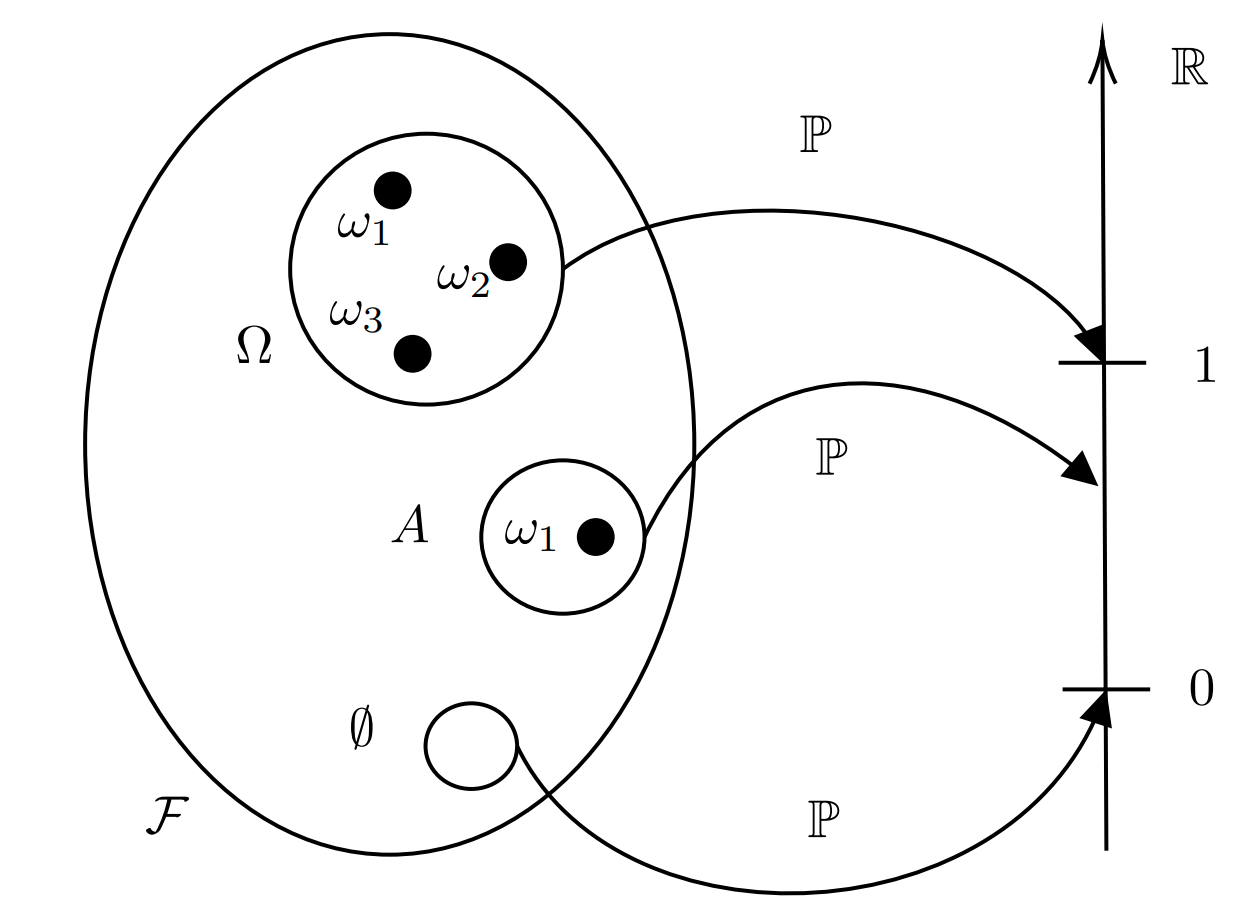We can see in the diagram above how the probability measure maps the empty set to zero, the sample space to $$1$$ and any other event to some number in $$[0, 1]$$.

# Random Variable

A random variable is also called a measurable function. Suppose you have two measurable spaces. One could be the pair of sample space and event space $$(\Omega, \mathcal{F})$$, and the other one could be some other arbitrary pair of a set and of its sigma algebra $$(\mathsf{E}, \mathcal{E})$$, although usually we choose $$\mathsf{E} = \mathbb{R}$$ and $$\mathcal{E} = \mathcal{B}(\mathbb{R})$$. Then a measurable function is a function that maps elements in $$\Omega$$ to elements in $$\mathsf{E}$$ with some additional properties. Notice how this function maps outcomes to elements of $$\mathsf{E}$$, it does not map events.

What property should this map satisfy? Imagine that we knew our sample space and event space, as in the coin tossing example. It might be a bit unhelpful to work on the actual sample space and event space, so instead we map them to alternative ones, that we are more comfortable using. For instance $$\mathbb{R}$$ and $$\mathcal{B}(\mathbb{R})$$. Then, it’s clear that we want our mapping to have a property that can guarantee us a sort of correspondence between the events in our original event space $$\mathcal{F}$$ and our transformed event space $$\mathcal{E}$$. For this reason, we require our measureable function, or random variable $X: \Omega \to \mathsf{E}$ to be such that the preimage $$X^{-1}(B)$$ of any $$\mathcal{E}$$-measurable set $$B\in\mathcal{E}$$ is a $$\mathcal{F}$$-measurable set. $X^{-1}(B) = \{\omega\in \Omega \, :\, X(\omega) \in B \} \in \mathcal{F} \qquad \forall \, B \in \mathcal{E}$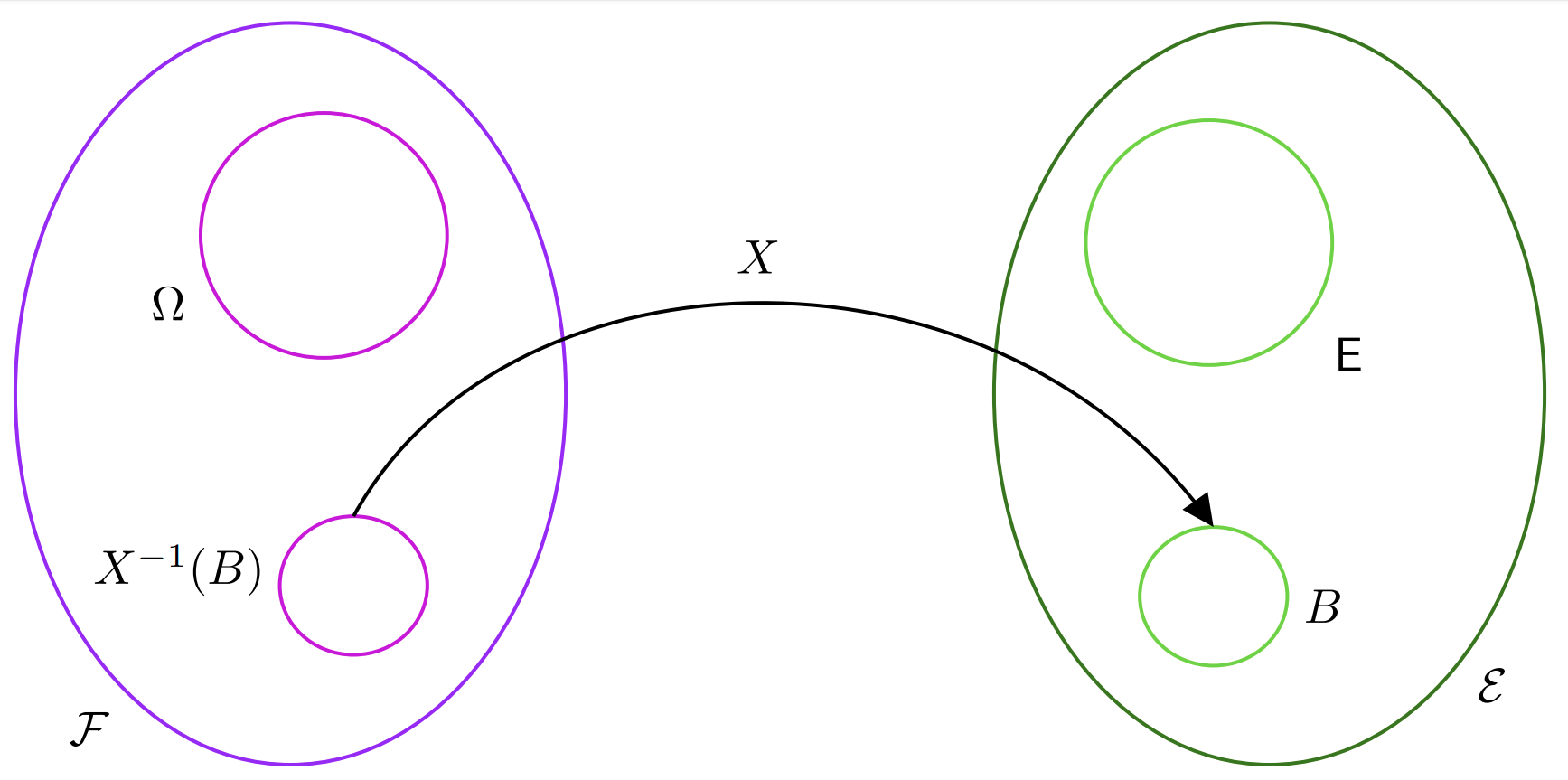In the diagram above we can see how the set $$B$$, which is an element of $$\mathcal{E}$$ has a pre-image, which we call $$X^{-1}(B)$$, which is an element of $$\mathcal{F}$$!

# Probability Distribution

A probability distribution is also called a pushforward measure of the probability measure $$\mathbb{P}$$ via the random variable $$X$$. Suppose we have a probability space $$(\Omega, \mathcal{F}, \mathbb{P})$$. This means we can assign probabilities to events in $$\mathcal{F}$$. Now suppose we have a measurable spae $$(\mathsf{E}, \mathcal{E})$$ but we don’t yet have a probability measure to measure events in it. How can we go about measuring sets in $$\mathcal{E}$$?

The key idea is that, given a set $$B$$ in $$\mathcal{E}$$, we can use a random variable $$X$$ to find the pre-image of such set in the event space $$\mathcal{F}$$ and then measure this set via the probability measure $$\mathcal{P}$$. This will then be our probability measurament also for $$B$$, as shown in the figure below.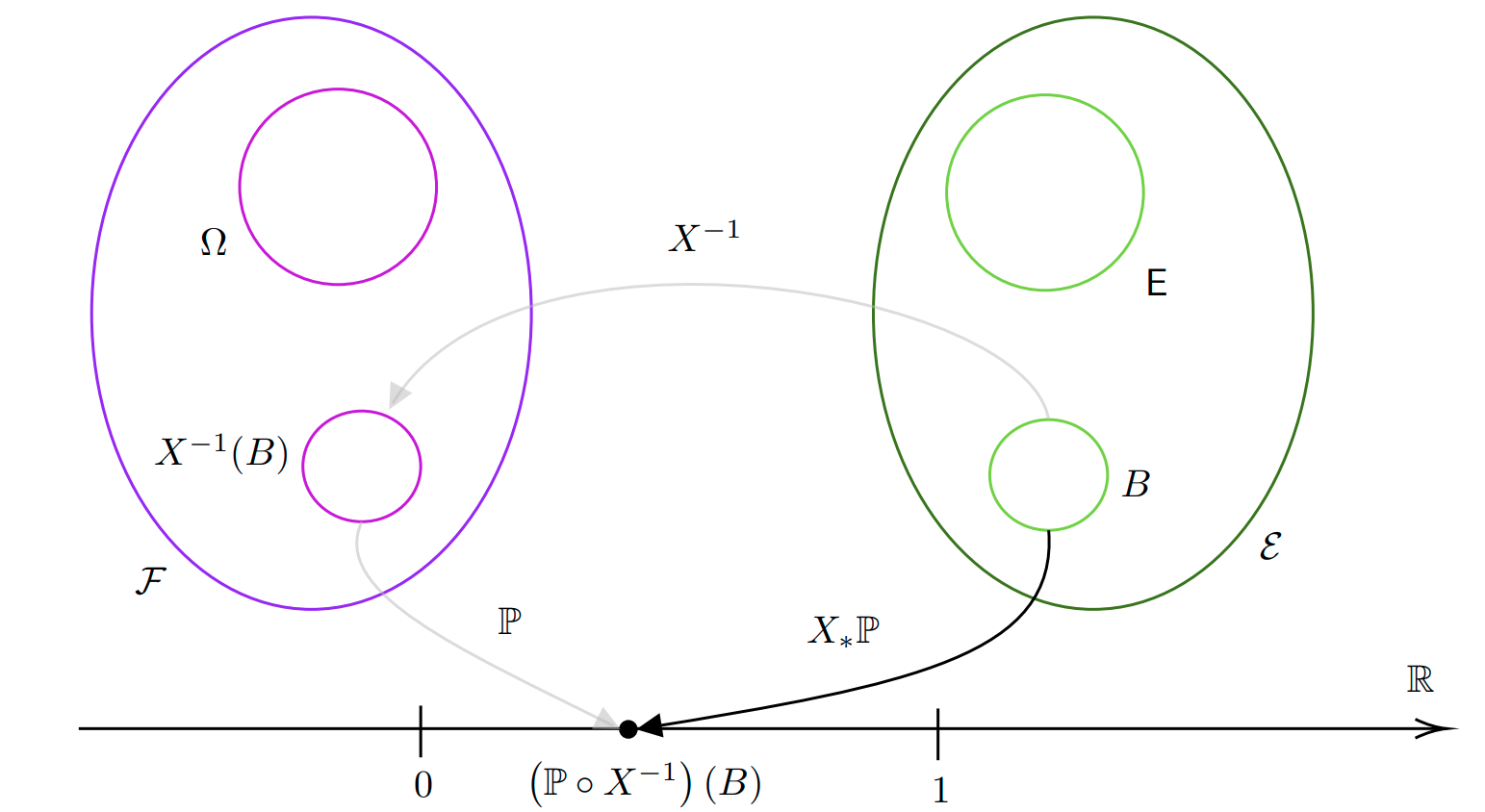The probability distribution, or pushforward, is then denoted as $$X_*\mathbb{P}$$ or $$\mathbb{P} \circ X^{-1}$$. The probability distribution is therefore a function mapping sets in $$\mathcal{E}$$ into $$[0, 1]$$: $X^*\mathbb{P}: \mathcal{E} \to [0, 1]$ In practice one often uses $$(\mathsf{E}, \mathcal{E}) = (\mathbb{R}^n, \mathcal{B}(\mathbb{R}^n))$$ so we have $X^*\mathbb{P}: \mathcal{B}(\mathbb{R}^n) \to [0, 1]$

# Probability Density Function

Consider $$(\mathbb{R}^n, \mathcal{B}(\mathbb{R}^n))$$. Suppose we have the usual Lebesgue measure $$\lambda^n$$ on such a measurable space. This would assign to each set $$A\in\mathcal{B}(\mathbb{R}^n)$$ our usual idea of size. However, now we have the random variable $$X$$ and we would like to “weight” the size of a set $$A\in\mathcal{B}(\mathbb{R}^n)$$ based on the “likelihood” of it happening. To do so, we assign a density to every point in $$\mathbb{R}^n$$ and to compute the new measure of $$A$$ according to $$X_*\mathbb{P}$$ we simply take the average of its density at all points in $$A$$ according to the Lebesgue measure $X_*\mathbb{P}(A) = \int_AdX_*\mathbb{P} = \int_A f d\lambda^n \qquad \forall A\in\mathcal{B}(\mathbb{R}^n)$ Essentially the density is almost like a derivative in the sense that it describes the rate of change of density of $$dX_*\mathbb{P}$$ with respect to $$\lambda^n$$. For this reason, we denote the density $$f$$ as follows $f = \frac{d X_*\mathbb{P}}{d \lambda^n}.$ This is called the Radon-Nikodym derivative. Using this notation is very convenient because we can use it to change the measure we are using to integrate. $X_*\mathbb{P}(A) = \int_A d X_*\mathbb{P} = \int_A \frac{dX_*\mathbb{P}}{d \lambda^n} d\lambda^n = \int_Af d\lambda^n$ The function $$f$$ is called the probability density function of the random variable $$X$$. Notice how one can express the probability distribution also in terms of the original probability measure $p(A) = X_*\mathbb{P}(A) = \int_A d\mathbb{P}\circ X^{-1} = \int_{X^{-1}(A)}d \mathbb{P}$

# Expectation, Change of Variable and Law of the Unconscious Statistician

The expectation of the random variable $$X$$ is given by the following Lebesgue integral $\mathbb{E}_{\mathbb{P}}[X] = \int_{\Omega} X d\mathbb{P} = \int_{\Omega}X(\omega) d\mathbb{P}(\omega)$ There is an important theorem called change of variable formula (i.e. LOTUS) that tells us that we can write the expectation of a tranformed random variable $$g \circ X: \Omega \to \mathbb{R}^n$$ where $$g:\mathbb{R}^n \to \mathbb{R}^n$$ in terms of the Lebesgue measure as follows $\mathbb{E}_{\mathbb{P}}[g \circ X] = \int_{\Omega} g \circ X \, d\mathbb{P} = \int_{\mathbb{R}^n} g \, dX_*\mathbb{P} = \int_{\mathbb{R}^n} g \frac{d X_*\mathbb{P}}{d\lambda^n} d\lambda^n = \int_{\mathbb{R}^n}g f \, d\lambda^n = \int_{\mathbb{R}^n} g(x) f(x) dx$ Importantly notice how the initial integral as over $$\Omega$$ and now it’s over $$X(\Omega) = \mathbb{R}^n$$.

# Markov Transition Kernel

Let $$(S, \mathcal{S})$$ and $$(T, \mathcal{T})$$ be two measurable spaces. A Markov Kernel is a map $K: S \times \mathcal{T} \to [0, 1]$ satisfying the following properties:

• $$K(x, \cdot): \mathcal{T} \to [0, 1]$$ is a probability measure for every $$x\in S$$. In practice this means that $$K(x, \cdot)$$ measures the probability of jumping from $$X\in S$$ to any point $$y\in A\in \mathcal{T}$$.
• $$K(\cdot, A): S\to [0,1]$$ is a measurable function. This means that given a set $$A$$ to jump to, the function that maps a point to the probability of jumping to any point in $$A$$ is measurable, i.e. a random variable.

The Markov Kernel defines two operators:

• It operates on the left with measures: Let $$\mu$$ be a measure on $$(S, \mathcal{S})$$. Then $$\mu K$$ is a measure on $$(T, \mathcal{T})$$ $\mu K(A) = \int_S \mu(dx) K(x, A) \qquad \qquad A\in\mathcal{T}$ Basically $$\mu K$$ gives you the average probability of jumping to some point $$y\in A$$ where $$A\in\mathcal{T}$$ if you start from point with measure $$\mu$$. Indeed, if we define $$K_A: S \to [0, 1]$$ as $$K_A(x) = K(x, A)$$ then we can write $\mu K(A) = \mathbb{E}_\mu[K_A] = \int_S K_A d\mu = \int_S K_A(x) d\mu(x) = \int_S K(x, A) \mu(dx)$

• It operates on the right with measurable functions

This is heavily influenced by https://www.randomservices.org/random/expect/Kernels.html.